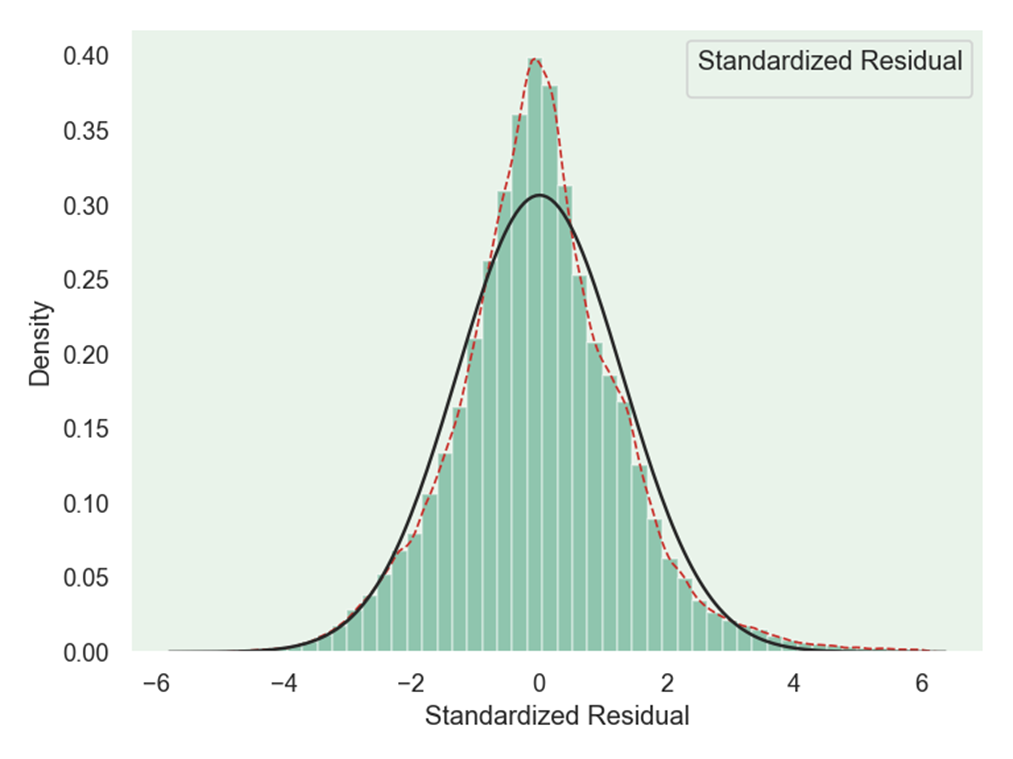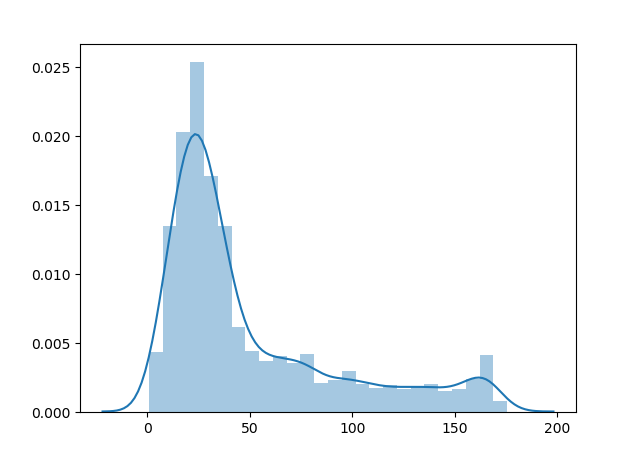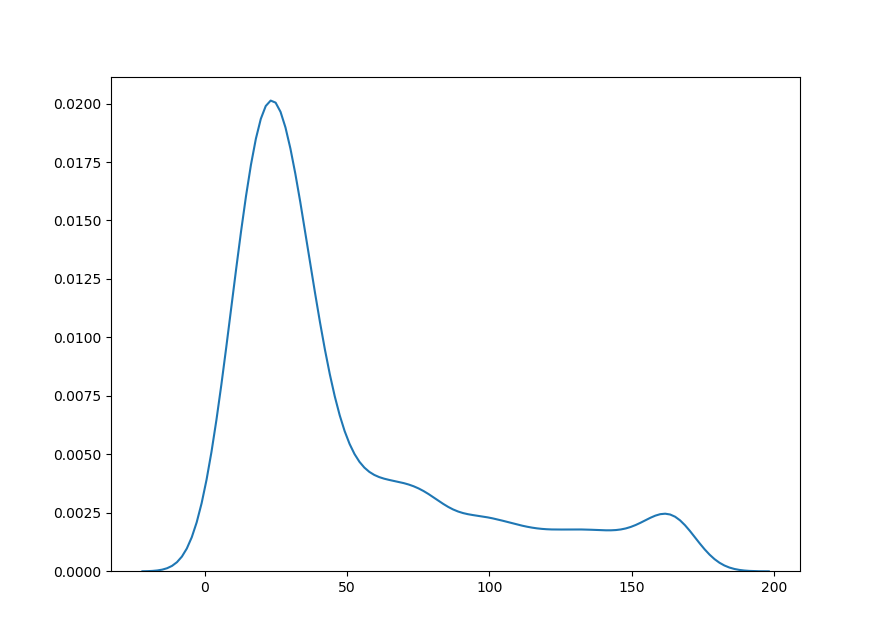• 2020-12-06 07:05:06

我正在研究一个由“脉冲长度”值和对应于每个脉冲长度的5或6个“电压值”组成的数据集。第一个值是脉冲长度，后面是电压。找到下表。在

15-56伏-47伏-53伏-50伏-50伏

16-49伏-46伏-52伏-47伏-50伏

47伏-50伏-50伏

18-50伏-51伏-48伏-48伏-45伏

19-49伏-51伏-45伏-47伏-52伏

20-45伏-47伏-50伏-47伏-54伏

21-46伏-52伏-52伏-49伏-54伏

22-53伏-51伏-53伏-56伏-52伏

23-52伏-45伏-51伏-56伏-53伏

24-51伏-52伏-54伏-58伏-52伏

25-56伏-53伏-57伏-55伏-53伏

26-53伏-52伏-55伏-52伏

27-54伏-49伏-56伏-54伏

28-52伏-52伏-57伏-56伏-53伏

29-63伏-60伏-54伏-58伏-61伏

30-59伏-70伏-61伏

X轴和Y轴是脉冲长度和电压，Z轴是它的概率分布。我有一个二维图，用的是一组“电压值”和它的概率。在图中，红色图形对应一个脉冲长度，绿色图形对应另一个脉冲长度。我用堆栈溢出(Plot normal distribution in 3D)中的一个多元正态分布示例，以同样的方式绘制3D图。由于我对3D绘图经验不足，我无法在同一个曲面上用不同的Y轴“脉冲长度”值绘制多个曲面图。我尝试的代码如下所示。在import numpy as np

import matplotlib

import matplotlib.pyplot as plt

from matplotlib.mlab import bivariate_normal

from mpl_toolkits.mplot3d import Axes3D

#Parameters to set

mu_x = -48.8

sigma_x = np.sqrt(6.5)

mu_y = 0

sigma_y = np.sqrt(16)

#Create grid and multivariate normal

x = range(-100,0)

y = range(15,30)

X, Y = np.meshgrid(x,y)

Z = bivariate_normal(X,Y,sigma_x,sigma_y,mu_x,mu_y)

#Make a 3D plot

fig = plt.figure()

ax = fig.gca(projection='3d')

ax.plot_surface(X, Y, Z,cmap='Reds',linewidth=0, antialiased=True,

zorder = 0.5)

ax.set_xlabel('Voltage')

ax.set_ylabel('Pulse Length')

ax.set_zlabel('Normal Distribution')

plt.show()

如果有人能帮我做同样的多脉冲长度，我会非常感激。

谢谢您。在

更多相关内容
• 目录   1、scipy库中各分布对应的方法   2、stats中各分布的常用方法及其功能   3、正态分布概率密度函数及其图象 ...    3）python绘制t分布和正态分布概率密度函数对比   6、F分布概率密度函数
• 主要介绍了python 计算概率密度、累计分布、逆函数的例子,具有很好的参考价值，希望对大家有所帮助。一起跟随小编过来看看吧
• 当我们想要弄清楚变量的统计特性时，往往想知道它是服从什么分布的，这时候就需要绘制概率分布直方 ...在seaborn中的distplot函数可以完成概率分布直方密度图的绘制 seaborn.distplot(a, bins=None, his.

当我们想要弄清楚变量的统计特性时，往往想知道它是服从什么分布的，这时候就需要绘制概率分布直方图

在python中我们可以使用seaborn库来进行绘制：

Seaborn是一个基于matplotlib的Python数据可视化库。它为绘制有吸引力和信息丰富的统计图形提供了高级界面。

首先需要导入seaborn库：

import seaborn as sns


在seaborn中的distplot函数可以完成概率分布直方图和密度图的绘制

seaborn.distplot(a, bins=None, hist=True, kde=True, rug=False,
fit=None, hist_kws=None, kde_kws=None, rug_kws=None, fit_kws=None,
color=None, vertical=False, norm_hist=False, axlabel=None,
label=None, ax=None)


下面直接给出我绘制时用到的代码：

import seaborn as sns
import matplotlib as mpl
import matplotlib.pyplot as plt
from scipy.stats import norm
from scipy.stats import laplace

txt=[]
data=[]
path = r"E:\rtklib\rtklib-test1\stav.txt"

#mpl.rc("figure", figsize=(9, 5))
with open(path, "r") as f:

for i in range(len(txt)):
data.append(float(txt[i].strip().split(',')))

sns.set_palette("hls")
#sns.set_style("whitegrid")
plt.figure(dpi=120)
sns.set(style='dark')
sns.set_style("dark", {"axes.facecolor": "#e9f3ea"})
g = sns.distplot(data,
hist=True,
kde=True,  # 开启核密度曲线kernel density estimate (KDE)
kde_kws={'linestyle': '--', 'linewidth': '1', 'color': '#c72e29',
# 设置外框线属性
},
fit=norm,
color='#098154',
axlabel='Standardized Residual',  # 设置x轴标题

)

plt.show()


其中，distplot的参数中，kde表示是否绘制核密度曲线；fit是选择拟合的分布，来分析数据究竟是符合什么分布，seaborn中提供了很多分布，可以在这里找到，使用时如上我的代码中导入的那样就可以：连续分布类型
最后的效果图如下：绘制过程中没有加入太多修饰，如果想要好看一点，可以修改一些参数：
其中一个有设置背景 的风格，主要有以下几种：

sns.set_style("whitegrid")
sns.set_style("dark")
sns.set_style("darkgrid")
sns.set_style("white")
sns.set_style("ticks")

展开全文算法
• 关于Python绘制正态分布图概率密度分布）以及遇到的问题（label无法显示） 目的：统计神经网络模型的参数分布情况 使用seaborn给我们提供的distplot函数来绘制，即调用sns.distplot()，并传入相关参数即可，这里...

# 关于Python绘制正态分布图（概率密度分布）以及遇到的问题（label无法显示）

目的：统计神经网络模型的参数分布情况

使用seaborn给我们提供的distplot函数来绘制，即调用sns.distplot()，并传入相关参数即可，这里我们用np.random.normal()函数来生成10000个均值为0，方差为1的数据，并将其传入sns.distplot()

from matplotlib import pyplot as plt
from scipy.stats import norm
import seaborn as sns
import numpy as np

w = np.random.normal(0, 1, 10000)

with plt.style.context(['science', 'no-latex']): # 如果没有安装SciencePlot库，可以把这行去掉
sns.distplot(w, bins=100, fit=norm)
plt.title("honest parameters")
plt.savefig('./distribution.svg', format='svg', dpi=300)
plt.show()


运行后的结果:可以看到已经成功画出来啦！

这时候如果我们想对比诚实模型恶意模型的参数分布，并给其添加对应的标签，label="honest", label="attacker",代码如下：

from matplotlib import pyplot as plt
from scipy.stats import norm
import seaborn as sns
import numpy as np

w = np.random.normal(0, 1, 10000)

with plt.style.context(['science', 'no-latex']): # 如果没有安装SciencePlot库，可以把这行去掉
sns.distplot(w, bins=100, fit=norm, label="honest")
plt.savefig('./distribution.svg', format='svg', dpi=300)
plt.show()


画出来是这样的:可以看到诚实模型恶意模型的参数分布已经绘制出来，但似乎设置的标签不起作用label="honest", label="attacker"，在图中无法显示。

解决方法：在plt.show()之前调用plt.legend()即可解决此问题

from matplotlib import pyplot as plt
from scipy.stats import norm
import seaborn as sns
import numpy as np

w = np.random.normal(0, 1, 10000)

with plt.style.context(['science', 'no-latex']): # 如果没有安装SciencePlot库，可以把这行去掉
sns.distplot(w, bins=100, fit=norm, label="honest")
plt.savefig('./distribution.svg', format='svg', dpi=300)
plt.legend() # 这行没有则无法显示标签
plt.show()


绘制结果如下，可以看到，已经能够正常显示label标签了：展开全文数据挖掘 深度学习
• kde（核密度估计）是对未知分布的估计，所以可能有超出去值范围的估计（比如下我定义的有效范围是0-180，但kde在<0和>180时仍然有曲线）。只要看自己想要的范围就好了，它在上面的拟合是可以的。 当然，也...

安装：直接pip

kde（核密度估计）是对未知分布的估计，所以可能有超出去值范围的估计（比如下图我定义的有效范围是0-180，但kde在<0和>180时仍然有曲线）。只要看自己想要的范围就好了，它在上面的拟合是可以的。

当然，也可以在distplot中用不同曲线拟合。

import seaborn as sns
from scipy import stats

# 绘制概率分布条形图，此时kde默认为true
sns.distplot(data, rug=False, hist=True)
# 也可以这样画kde
# hist=False表示不要bar
# 这里用gamma分布拟合
sns.distplot(data, hist=False, kde=False, fit=stats.gamma)展开全文• matlab作概率密度函数很简单，但是本人学的python，因此想用python作图，从txt文本中读取数据，然后用python概率密度函数图像，
• Python绘制概率分布直方 文章目录Python绘制概率分布直方一、代码1、案例一2、案例二二、运行效果1、案例一2、案例二 一、代码 1、案例一 import random import numpy as np import matplotlib.pyplot as plt X...深度学习 机器学习
• 文章目录术语前言整数浮点数抽取字节洗牌排列贝塔分布二项分布卡方分布狄利克雷分布指数分布F分布伽玛分布几何分布耿贝尔分布超几何分布拉普拉斯分布（双指数分布）逻辑斯谛分布正态分布（高斯分布）对数正态分布...
• for i in sorted(list(set(df['yearmonth']))): df_sort = df[df['yearmonth'] == i].groupby('cust_id')['y','pred'].sum().sort_values('y',ascending = False) df_sort['yearmonth'] = i ...
• beta分布的最大特点是其多样性, 从下可以看出, beta分布具有各种形态, 有U形, 类似正态分布的形状, 类似uniform分布的形状等, 正式这一特质使beta分布在共轭先验的计算中起到重要作用: import matplotlib.pyplot ...
• 对于pandas的dataframe，...对于pandas的dataframe，绘制概率密度图方法如下： //pdf是pandas的dataframe, delta_time是其中一列 pdf.delta_time.dropna().plot(kind='kde', xlim=(-50,300)) 以上这篇python绘制直方
• python 服从正态分布概率密度函数和累积密度函数 服从正太分布下,概率密度函数公式 公式解释: f(x): 是某样本(样本以数值形式表现)为某数值时发生的概率 0<f(x)<1 x: 是随机抽样的数值,取值范围从负...
• 概率密度函数为正态分布的期望值μ决定了其位置，其标准差σ决定了分布的幅度。当μ = 0,σ = 1时的正态分布是标准正态分布。 正态曲线呈钟型，两头低，中间高，左右对称因其曲线呈钟形，因此人们又经常称之为...
• 概率分布表示样本数据的模样，长的好不好看如果有图像展示出来就非常完美了，使用Python绘制频率分布直方非常简洁，因为用的频次非常高，这里记录下来。还是Python大法好，代码简洁不拖沓~ 如果数据取值的范围跨度...
• mumpy 、random import numpy as np import seaborn as sns import matplotlib.pyplot as plt from scipy import stats np.random.seed(1234) rn1 = np.random.normal(loc = 0, scale = 1, size = 1000) ...
• 这篇文章将向您展示如何：使用高斯核估计2个分布的PDF(PDF即Probability Density Function，概率密度函数)使用Matplotlib表示PDF，其中密度周围带有标记的轮廓线如何提取轮廓线如何在3D(3维空间)中绘制上述高斯核...
• 编辑：Peter转自：机器之心机器学习有其独特的数学基础，我们用微积分来处理变化无限小的函数，并计算...与此同时，从更细的角度来说，随机变量的概率分布也是我们必须理解的内容。在这篇文章中，项目作者介绍了所...机器学习 人工智能 大数据
• Matplotlib在概率密度曲线的基础上绘制积分区域实例matplotlib
• 利用geopandas和已有地理空间数据，结合shp矢量文件绘制感兴趣区域的物种分布密度图
• 导语：大家好，我是小π！我的公众号是关于自己在数据分析/挖掘学习过程中的一些技术和总结分享，文章会持续更新......基本概念离散型随机变量如果随机变量X的所有...相应的概率分布有正态分布，均匀分布，指数分布...
• 之前一直想尝试着用Matplotlib绘制计算结果中的二维密度分布图，这样即省去了许多数据处理的麻烦，也方便直接在Linux系统中观察计算的结果。但对Numpy和Maltplotlib的熟练程度还不够，对于计算程序产生的非矩阵式的...
• 头大，外行人做个咋这么难，趋势线还没有研究出来怎么加上去，哎 import matplotlib.pyplot as plt from scipy.stats import gaussian_kde from mpl_toolkits.axes_grid1 import make_axes_locatable import ...
• 在这之外，可以由用户自己创建新的分布 针对连续变量的主要方法有： Rvs:随机变量 Pdf:概率密度函数 Cdf:累计分布函数 Sf:残差函数 Ppf:百分点函数，cdf/1 Isf:1/sf 不过..首先其文档对我这种六级低空飘过的人来说读...
• 计算分布函数(也称累积概率函数CDF) python代码如下： import numpy as np data = np.random.randn(200) # 随机生成一些数 # 一个公式即可计算出概率函数 cdf_value = 1. * np.arange(len(data)) / (len(data) - 1......